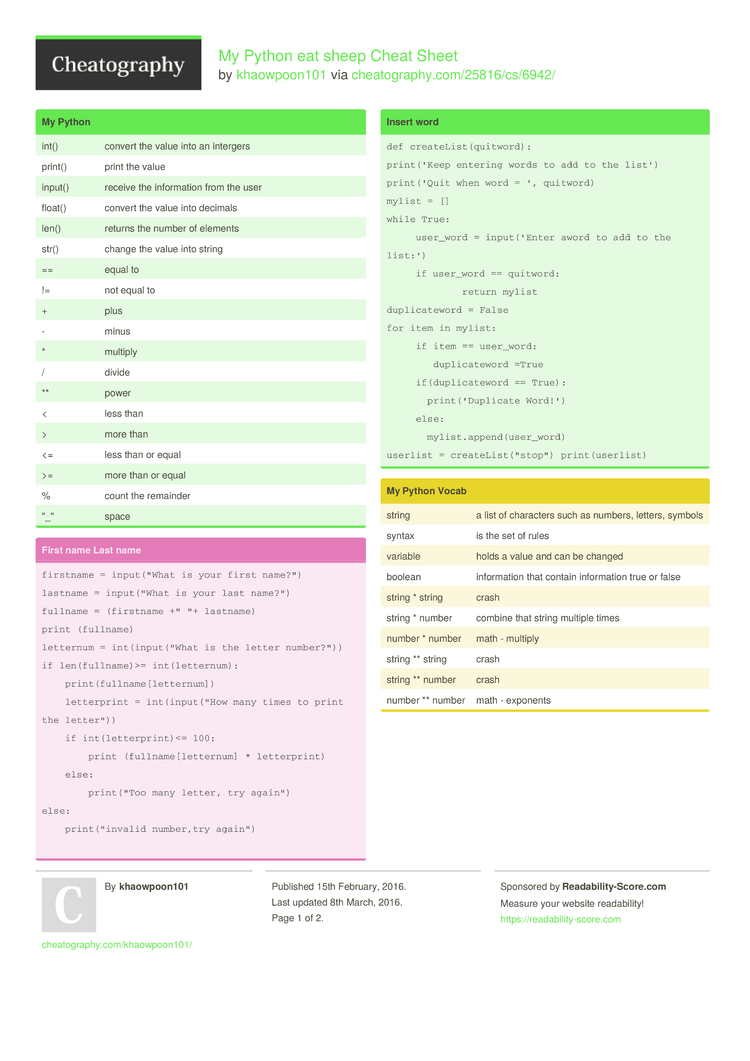# My Python eat sheep Cheat Sheet by khaowpoon101

### My Python

 int() convert the value into an intergers print() print the value input() receive the inform­ation from the user float() convert the value into decimals len() returns the number of elements str() change the value into string == equal to != not equal to + plus - minus * multiply / divide ** power < less than > more than <= less than or equal >= more than or equal % count the remainder "­_" space

### Insert word

 ```def createList(quitword): print('Keep entering words to add to the list') print('Quit when word = ', quitword) mylist = [] while True:      user_word = input('Enter aword to add to the list:')      if user_word == quitword:              return mylist duplicateword = False for item in mylist:      if item == user_word:         duplicateword =True      if(duplicateword == True):        print('Duplicate Word!')      else:        mylist.append(user_word) userlist = createList("stop") print(userlist)```

### First name Last name

 ```firstname = input("What is your first name?") lastname = input("What is your last name?") fullname = (firstname +" "+ lastname) print (fullname) letternum = int(input("What is the letter number?")) if len(fullname)>= int(letternum):     print(fullname[letternum])     letterprint = int(input("How many times to print the letter"))     if int(letterprint)<= 100:         print (fullname[letternum] * letterprint)     else:         print("Too many letter, try again") else:     print("invalid number,try again")```

### My Python Vocab

 string a list of characters such as numbers, letters, symbols syntax is the set of rules variable holds a value and can be changed boolean inform­ation that contain inform­ation true or false string * string crash string * number combine that string multiple times number * number math - multiply string ** string crash string ** number crash number ** number math - exponents

### Guessing game

 ```import random mylist = ['sodium','copper','platinum','gold','silver'] chance = 3 score = 0 print ("Guessing Game") while chance > 0:     print (mylist)     random_item = random.choice(mylist)     user_guess = input("Please guess a word in the list:")     if user_guess in mylist:         if(user_guess) == (random_item):             print ("That's correct")             score = int(score)+100             print ("Score:",score)             print ("Chance:",chance)         else:             print ("sorry, wrong choice")             chance = int (chance)-1             print ("score: ",score)             print ("chance: ",chance)             print (random_item)     else:            print ("sorry, that is not even in the list!")            print ("try again!")            print ("score: ",score)            chance = int(chance)-1            print ("chance: ",chance)            print ("your total score is: ",score)```

## Download the My Python eat sheep Cheat Sheet2 Pages
//media.cheatography.com/storage/thumb/khaowpoon101_my-python-eat-sheep.750.jpg

PDF (recommended)